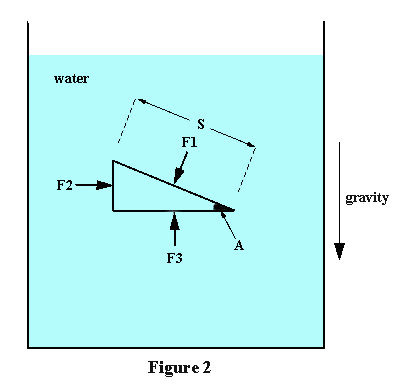# Hydrostatic Pressure at a Point

Every diver is taught that the pressure at any point in a still body of water is the sum of the pressure due to the weight of the atmosphere and the pressure due to the weight of the column of water directly above that point. That much is fairly straightforward. What may not be so obvious, however, is that the total pressure at a point is exactly the same from all directions.Assume static equilibrium, i.e. no part of the water is in motion relative to any other part. Lack of resistance to shear (property (i)) implies that the external force on each slab face must act in a direction perpendicular to that face. If this were not so, there would be a shear stress across the slab face and it would move, which would contradict the assumption of static equilibrium. The forces on the slab faces are therefore as shown in Figure 1. Not shown are the two other forces which act on the slab edges that are in the plane of the figure.`F2 = F1*sinA                   Equation 1`

`A1=L*S ; A2=L(S*sinA)          Equation 2`
Since pressure is force per unit area, we therefore have:

`F1 = P1*A1 =  P1*L*S  ;  F2 = P2*A2 = P2*L(S*sinA)          Equation 3`

`P1 = P2                        Equation 4`

`F1*cosA + Mg = F3              Equation 5`

`M = d[0.5L(S*sinA)(S*cosA)]    Equation 6`

`A3 = L(S*cosA)                 Equation 7`

`F3 = P3*L(S*cosA)              Equation 8`

`P3 = P1 + 0.5*d(S*sinA)g       Equation 9`

`P3 = P1                         Equation 10`